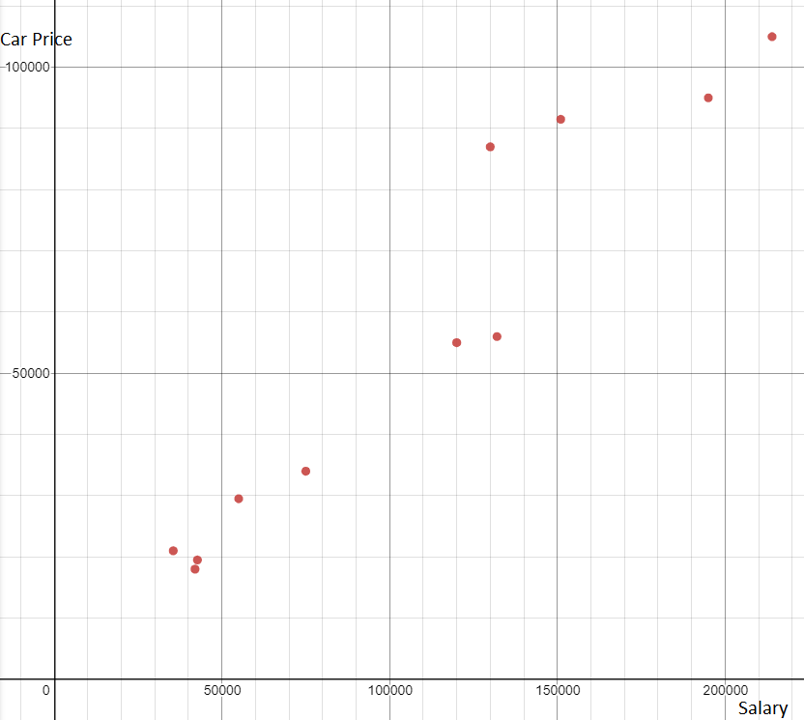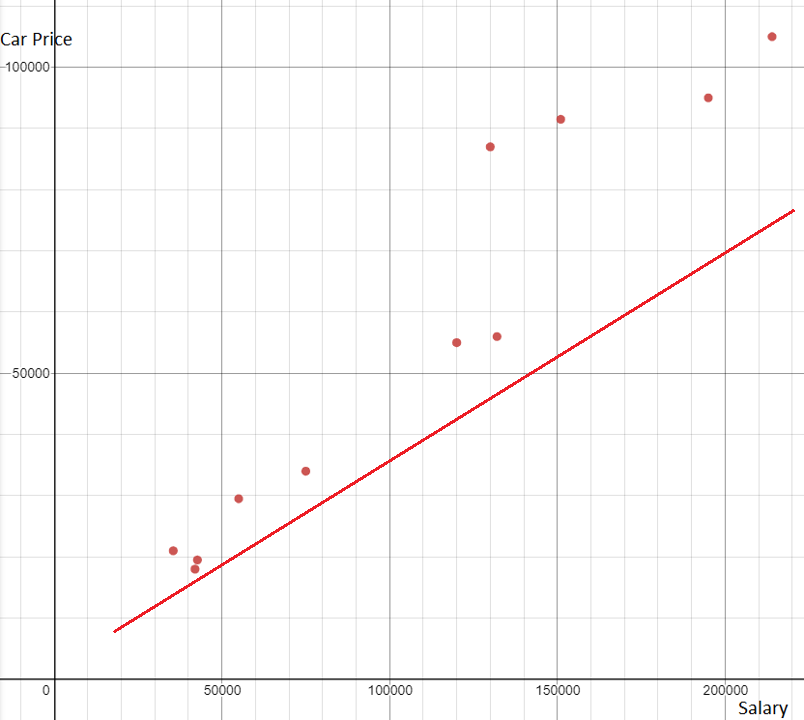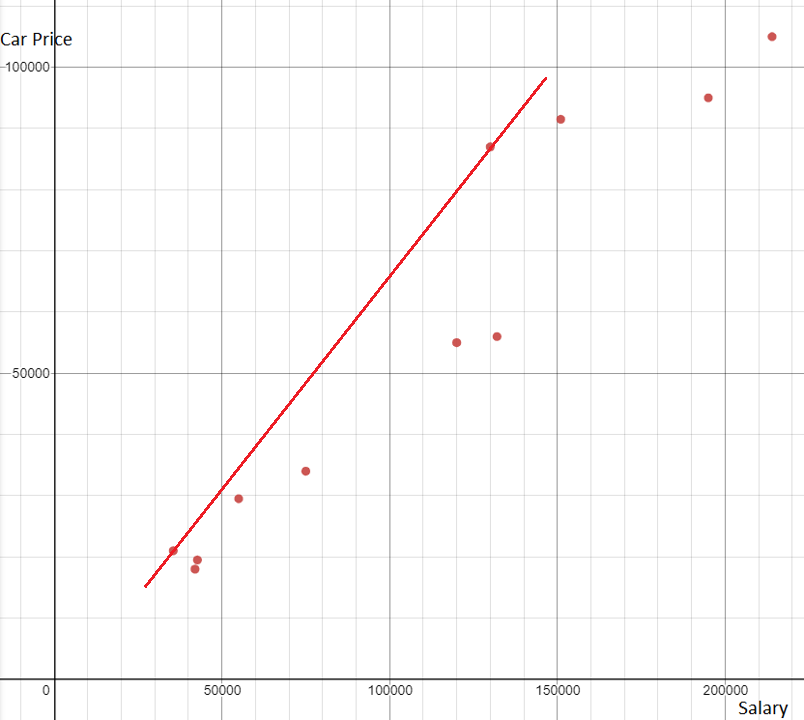# Interpolation and Extrapolation

We use graphs to visualize trends between variables. We can use the trend to make predictions about other data points not available. When the predictable data falls within the available data points it is called interpolation. When the data falls outside the available data points it is called extrapolation.

## Line of Best Fit

A line of best fit simply refers to a line drawn through a scatter plot of data points that best expresses the relationship between those points

To draw the line of best fit, we can estimated by drawing a line through most of the data points. However, there also exist a way to calculate the actual best line using Least Squares Regression (learnt later).

The table below shows the annual salaries of consumers and the price of cars they drive (in thousands of dollars).

 X (Salary, k$) Y (Car price, k$) 42.7 195 35.5 214 75 130 42 151 55 120 132 19.5 95 21 105 34 87 18 91.5 29.5 55 56

By plotting the points on a graph, we see there is a linear positive relation between the salary and cost of the car. That means, in general, someone who makes more money will have a more expensive car.

We can draw a line of best fit through the data that shows the trend between the two variables. The line should go through many points and be close to the others.Let's look at some not so good lines of best fit. The first line doesn't go through any of the points. The second line goes through two points but is far away from the others.

A tip for drawing a good line of best fit is to make sure the number of points above and below the line are similar.From the above graph, write the equation of the line for the line of best fit.?